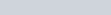ChemTalk

# Arrhenius Equation### Arrhenius equation- Core Concept

In this tutorial, you will learn what the Arrhenius equation is, how to use the equation to determine the activation energy or rate constant of a reaction, and how to derive it. Using the Arrhenius Equation is an important concept in chemical kinetics.

#### What is the Arrhenius equation?

The Arrhenius equation is used to determine the activation energy or rate constant of a chemical reaction. as the temperature changes. If you want to see how the rate constant changes when the temperature changes, the Arrhenius equation is your friend.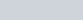Where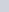is rate constant,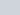is the energy of activation (energy required to get the reaction started),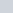is the frequency factor,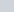is the gas constant, and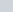is temperature.

#### Derivation of the Arrhenius equation

The Arrhenius equation can be derived from experimental data. When linearizing the equation into the form of y=mx+b, we get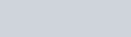Now we want to solve for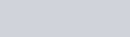Raise both sides to the e to get rid of the natural log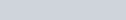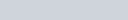When we are given two temperature inputs, we can use a different form of the equation.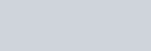#### Visualizing the Arrhenius Equation

By plotting the previous equation, we can find attributes of a reaction. For example, graphing the linearized function by plotting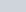vs.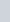, you can determine the slope which will be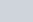and the Arrhenius constant, which will be the y-intercept.

### Example Problems of the Arrhenius Equation:

The rate constant k of a chemical reaction is measured at two different temperatures: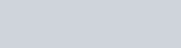Calculate the energy of activation for this chemical reaction.

#### Solution:

Since we are given two temperature inputs, we must use the second form of the equation:First, we convert the Celsius temperatures to Kelvin by adding 273.15: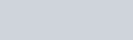Now let’s plug in all the values. We know that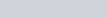andand now we have the T values.### Example problem 2: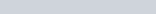The above decomposition reaction has an activation energy of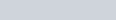and a rate constant of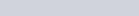at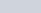. At what temperature will the rate be twice as that of.

If you are asked to solve for a different variable, there are a few ways to check your work. The larger rate constant k should always be associated with the higher temperature. The activation energy should always have a positive sign. The reaction rate and rate constant are both larger at higher temperatures so long as all other factors are held constant.

#### Solution

If the reaction rate is twice as fast,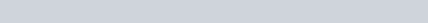• A graph of data used to generate the Arrhenius equation is called an Arrhenius plot, where the X axis is, and the Y axis is the natural log of k. The slope is.
• The equation is named after the Swedish chemist Svante Arrhenius who discovered the relationship between reaction rates and temperature in 1889.

## Arrhenius Equation Practice Problems

Problem 1

At a temperature of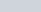, you measure a certain reaction to have a certain rate constant (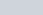). You increase the temperature toand observe that the rate constant has tripled (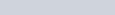). What is the reaction’s activation energy?

Problem 2

At a temperature of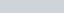, you observe a reaction to have a rate constant of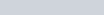and an activation energy of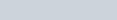. Calculate the frequency factor.

## Arrhenius Equation Practice Problem Solution

1:2: